# 逻辑斯蒂回归(Logistic Regression)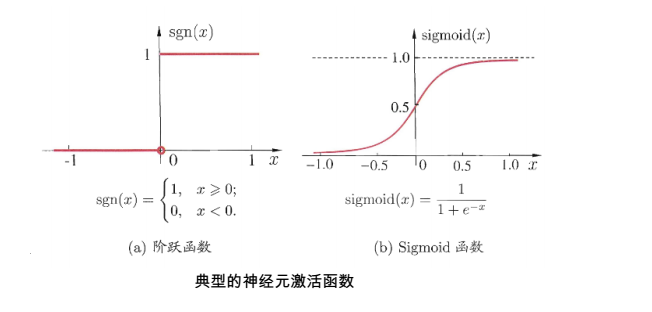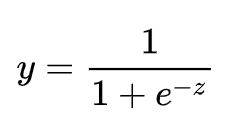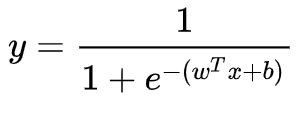# 损失函数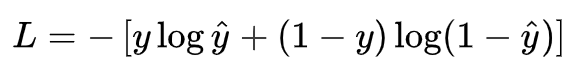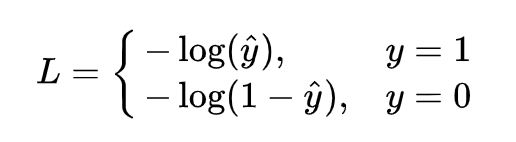# 优化求解

LR的学习任务转化为数学的优化形式为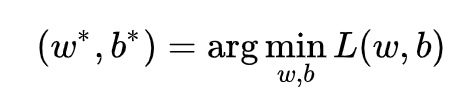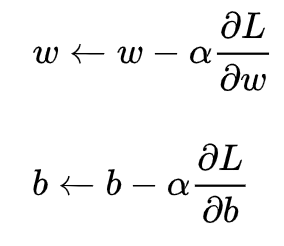Newton Method（牛顿法）

Quasi-Newton Method(拟牛顿法)

BFGS Method

L-BFGS(Limited-memory BFGS)

# 自主实现

# -*- coding: utf-8 -*-

import numpy as np

class LogisticRegression(object):

def __init__(self, learning_rate=0.1, max_iter=100, seed=None):
self.seed = seed
self.lr = learning_rate
self.max_iter = max_iter

def fit(self, x, y):
np.random.seed(self.seed)
self.w = np.random.normal(loc=0.0, scale=1.0, size=x.shape)
self.b = np.random.normal(loc=0.0, scale=1.0)
self.x = x
self.y = y
for i in range(self.max_iter):
self._update_step()
# print('loss: \t{}'.format(self.loss()))
# print('score: \t{}'.format(self.score()))
# print('w: \t{}'.format(self.w))
# print('b: \t{}'.format(self.b))

def _sigmoid(self, z):
return 1.0 / (1.0 + np.exp(-z))

def _f(self, x, w, b):
z = x.dot(w) + b
return self._sigmoid(z)

def predict_proba(self, x=None):
if x is None:
x = self.x
y_pred = self._f(x, self.w, self.b)
return y_pred

def predict(self, x=None):
if x is None:
x = self.x
y_pred_proba = self._f(x, self.w, self.b)
y_pred = np.array([0 if y_pred_proba[i] < 0.5 else 1 for i in range(len(y_pred_proba))])
return y_pred

def score(self, y_true=None, y_pred=None):
if y_true is None or y_pred is None:
y_true = self.y
y_pred = self.predict()
acc = np.mean([1 if y_true[i] == y_pred[i] else 0 for i in range(len(y_true))])
return acc

def loss(self, y_true=None, y_pred_proba=None):
if y_true is None or y_pred_proba is None:
y_true = self.y
y_pred_proba = self.predict_proba()
return np.mean(-1.0 * (y_true * np.log(y_pred_proba) + (1.0 - y_true) * np.log(1.0 - y_pred_proba)))

y_pred = self.predict()
d_w = (y_pred - self.y).dot(self.x) / len(self.y)
d_b = np.mean(y_pred - self.y)
return d_w, d_b

def _update_step(self):
self.w = self.w - self.lr * d_w
self.b = self.b - self.lr * d_b
return self.w, self.b


# -*- coding: utf-8 -*-

import numpy as np

def generate_data(seed):
np.random.seed(seed)
data_size_1 = 300
x1_1 = np.random.normal(loc=5.0, scale=1.0, size=data_size_1)
x2_1 = np.random.normal(loc=4.0, scale=1.0, size=data_size_1)
y_1 = [0 for _ in range(data_size_1)]
data_size_2 = 400
x1_2 = np.random.normal(loc=10.0, scale=2.0, size=data_size_2)
x2_2 = np.random.normal(loc=8.0, scale=2.0, size=data_size_2)
y_2 = [1 for _ in range(data_size_2)]
x1 = np.concatenate((x1_1, x1_2), axis=0)
x2 = np.concatenate((x2_1, x2_2), axis=0)
x = np.hstack((x1.reshape(-1,1), x2.reshape(-1,1)))
y = np.concatenate((y_1, y_2), axis=0)
data_size_all = data_size_1+data_size_2
shuffled_index = np.random.permutation(data_size_all)
x = x[shuffled_index]
y = y[shuffled_index]
return x, y

def train_test_split(x, y):
split_index = int(len(y)*0.7)
x_train = x[:split_index]
y_train = y[:split_index]
x_test = x[split_index:]
y_test = y[split_index:]
return x_train, y_train, x_test, y_test


# -*- coding: utf-8 -*-

import numpy as np
import matplotlib.pyplot as plt
import data_helper
from logistic_regression import *

# data generation
x, y = data_helper.generate_data(seed=272)
x_train, y_train, x_test, y_test = data_helper.train_test_split(x, y)

# visualize data
# plt.scatter(x_train[:,0], x_train[:,1], c=y_train, marker='.')
# plt.show()
# plt.scatter(x_test[:,0], x_test[:,1], c=y_test, marker='.')
# plt.show()

# data normalization
x_train = (x_train - np.min(x_train, axis=0)) / (np.max(x_train, axis=0) - np.min(x_train, axis=0))
x_test = (x_test - np.min(x_test, axis=0)) / (np.max(x_test, axis=0) - np.min(x_test, axis=0))

# Logistic regression classifier
clf = LogisticRegression(learning_rate=0.1, max_iter=500, seed=272)
clf.fit(x_train, y_train)

# plot the result
split_boundary_func = lambda x: (-clf.b - clf.w * x) / clf.w
xx = np.arange(0.1, 0.6, 0.1)
plt.scatter(x_train[:,0], x_train[:,1], c=y_train, marker='.')
plt.plot(xx, split_boundary_func(xx), c='red')
plt.show()

# loss on test set
y_test_pred = clf.predict(x_test)
y_test_pred_proba = clf.predict_proba(x_test)
print(clf.score(y_test, y_test_pred))
print(clf.loss(y_test, y_test_pred_proba))
# print(y_test_pred_proba)


# sklearn

sklearn.linear_model模块提供了很多模型供我们使用，比如Logistic回归、Lasso回归、贝叶斯脊回归等，可见需要学习的东西还有很多很多。

LogisticRegression这个函数，一共有14个参数，详见https://scikit-learn.org/dev/modules/generated/sklearn.linear_model.LogisticRegression.html

# LR 的优缺点

## LR的优点

1、形式简单，模型的可解释性非常好。从特征的权重可以看到不同的特征对最后结果的影响，某个特征的权重值比较高，那么这个特征最后对结果的影响会比较大。

2、模型效果不错。在工程上是可以接受的（作为baseline)，如果特征工程做的好，效果不会太差，并且特征工程可以大家并行开发，大大加快开发的速度。

3、训练速度较快。分类的时候，计算量仅仅只和特征的数目相关。并且逻辑回归的分布式优化sgd发展比较成熟，训练的速度可以通过堆机器进一步提高，这样我们可以在短时间内迭代好几个版本的模型。

4、资源占用小,尤其是内存。因为只需要存储各个维度的特征值，。

5、方便输出结果调整。逻辑回归可以很方便的得到最后的分类结果，因为输出的是每个样本的概率分数，我们可以很容易的对这些概率分数进行cutoff，也就是划分阈值(大于某个阈值的是一类，小于某个阈值的是一类)。

## LR的缺点

1、准确率并不是很高。因为形式非常的简单(非常类似线性模型)，很难去拟合数据的真实分布。

2、很难处理数据不平衡的问题。举个例子：如果我们对于一个正负样本非常不平衡的问题比如正负样本比 10000:1.我们把所有样本都预测为正也能使损失函数的值比较小。但是作为一个分类器，它对正负样本的区分能力不会很好。

3、处理非线性数据较麻烦。逻辑回归在不引入其他方法的情况下，只能处理线性可分的数据，或者进一步说，处理二分类的问题 。

4、逻辑回归本身无法筛选特征。有时候，我们会用gbdt来筛选特征，然后再上逻辑回归。

# LR将连续特征离散化的原因

1、稀疏向量内积乘法运算速度快，计算结果方便存储，容易scalable（扩展）。

2、离散化后的特征对异常数据有很强的鲁棒性：比如一个特征是年龄>30是1，否则0。如果特征没有离散化，一个异常数据“年龄300岁”会给模型造成很大的干扰。

3、逻辑回归属于广义线性模型，表达能力受限；单变量离散化为N个后，每个变量有单独的权重，相当于为模型引入了非线性，能够提升模型表达能力，加大拟合。

4、离散化后可以进行特征交叉，由M+N个变量变为M*N个变量，进一步引入非线性，提升表达能力。

5、特征离散化后，模型会更稳定，比如如果对用户年龄离散化，20-30作为一个区间，不会因为一个用户年龄长了一岁就变成一个完全不同的人。当然处于区间相邻处的样本会刚好相反，所以怎么划分区间是门学问。

# 逻辑回归和线性回归的区别和联系

1）线性回归要求因变量服从正态分布，logistic回归对变量分布没有要求。

2）线性回归要求因变量（Y）是连续性数值变量，而logistic回归要求因变量是分类型变量。

3）线性回归要求自变量和因变量呈线性关系，而logistic回归不要求自变量和因变量呈线性关系

4）线性回归是直接分析因变量与自变量的关系，而logistic回归是分析因变量取某个值的概率与自变量的关系

1、回归与分类：回归模型就是预测一个连续变量(如降水量，价格等)。在分类问题中，预测属于某类的概率，可以看成回归问题。这可以说是使用回归算法的分类方法。

2、输出：直接使用线性回归的输出作为概率是有问题的，因为其值有可能小于0或者大于1,这是不符合实际情况的，逻辑回归的输出正是[0,1]区间

3、参数估计方法：

1）线性回归中使用的是最小化平方误差损失函数，对偏离真实值越远的数据惩罚越严重。这样做会有什么问题呢？假如使用线性回归对{0,1}二分类问题做预测，则一个真值为1的样本，其预测值为50，那么将会对其产生很大的惩罚，这也和实际情况不符合，更大的预测值说明为1的可能性越大，而不应该惩罚的越严重。

2）逻辑回归使用对数似然函数进行参数估计，使用交叉熵作为损失函数，对预测错误的惩罚是随着输出的增大，逐渐逼近一个常数，这就不存在上述问题了

3）也正是因为使用的参数估计的方法不同，线性回归模型更容易受到异常值(outlier)的影响，有可能需要不断变换阈值(threshold)。

# LR和SVM的关系

1、LR和SVM都可以处理分类问题，且一般都用于处理线性二分类问题（在改进的情况下可以处理多分类问题）

2、两个方法都可以增加不同的正则化项，如l1、l2等等。所以在很多实验中，两种算法的结果是很接近的。

1、LR是参数模型，SVM是非参数模型。

2、从目标函数来看，区别在于逻辑回归采用的是logistical loss，SVM采用的是hinge loss，这两个损失函数的目的都是增加对分类影响较大的数据点的权重，减少与分类关系较小的数据点的权重。

3、SVM的处理方法是只考虑support vectors，也就是和分类最相关的少数点，去学习分类器。而逻辑回归通过非线性映射，大大减小了离分类平面较远的点的权重，相对提升了与分类最相关的数据点的权重。

4、逻辑回归相对来说模型更简单，好理解，特别是大规模线性分类时比较方便。而SVM的理解和优化相对来说复杂一些，SVM转化为对偶问题后,分类只需要计算与少数几个支持向量的距离,这个在进行复杂核函数计算时优势很明显,能够大大简化模型和计算。

5、logic 能做的 svm能做，但可能在准确率上有问题，svm能做的logic有的做不了。

# 参考资料

https://xueqiu.com/8566534281/145350684?page=2

https://blog.csdn.net/qq_32742009/article/details/81516140

 [机器学习 算法笔记- 线性回归（Linear Regression）](https://www.cnblogs.com/geo-will/p/10468253.html)

https://www.cnblogs.com/yanghh/p/13697116.html

https://zhuanlan.zhihu.com/p/41458511